Next: Progressive Alignment:

# Near Optimal Multiple Sequence Alignments Using a Traveling Salesman Problem Approach

Chantal Korostensky and Gaston Gonnet
Swiss Federal Institute of Technology
Institute of Scientific Computing
ETH Zürich, Switzerland
korosten, gonnet@inf.ethz.ch

### Abstract:

We present a new method for the calculation of multiple sequence alignments (MSAs). The input to our problem are n protein sequences. We assume that the sequences are related with each other and that there exists some unknown evolutionary tree that corresponds to the MSA. One advantage of our method is that the scoring can be done with reference to this phylogenetic tree, even though the tree structure itself may remain unknown. Instead of computing an evolutionary tree, we only need to compute a circular tour of the tree which is determined via a Traveling Salesman Problem (TSP) algorithm. Our algorithm can calculate a near optimal MSA and has a performance guarantee of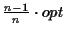(where opt is the optimal score of the MSA). The algorithm runs in O(k2 n2) time, where k is the length of the longest input sequence. From there we improve the alignment further. Experimental results are shown at the end.

Introduction The computation of multiple sequence alignments (MSAs) remains one of the major open problems in computational biology. Possibly this is due to the complexity as most problems associated with MSAs are NP-complete . It is therefore desirable to develop heuristics that try to come as close to the optimum as possible but terminate within reasonable time and space bounds. An MSA consists of a set of strings, where each string represents an amino acid, DNA or RNA sequence (see Definition 1.1).

Figure: The sequences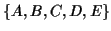of the MSA on the left are related by the evolutionary tree on the right.
  A: RPCVCP___VLRQAAQ__QVLQRQIIQGPQQLRRLF_AA B: RPCACP___VLRQVVQ__QALQRQIIQGPQQLRRLF_AA C: KPCLCPKQAAVKQAAH__QQLYQGQLQGPKQVRRAFRLL D: KPCVCPRQLVLRQAAHLAQQLYQGQ____RQVRRAF_VA E: KPCVCPRQLVLRQAAH__QQLYQGQ____RQVRRLF_AA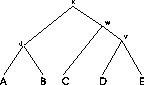Example: In the MSA in Figure 1 five sequences are aligned (n=5). We assume that the sequences are related and that we have the correct tree. The internal nodes represent unknown ancestor sequences.
Definitions

Definition 0.1   Given is a set of sequences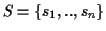with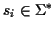whereis a finite alphabet. A Multiple Sequence Alignment (MSA) consists of a set of sequences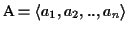with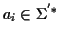where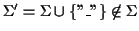.. The sequence obtained from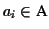by removing allgap characters is equal to si.

Definition 0.2   The characteror any contiguous sequence ofwithin an aligned sequenceis called a gap. A gap g has length len(g), where the length is the number of contiguous dashes. It starts at a position pos(g) in sequenceand ends at position end(g).

Definition 0.3   An MSA scoring function is a function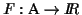.

Definition 0.4   Let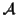be the set of all possible MSAs that can be generated for a given set of sequences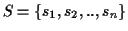. The optimal MSA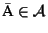is an MSA such that w.l.o.g.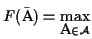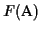. In many scoring functions the minimum is the optimal.

Problem 0.1 (MSA problem)   Given is a set of sequences. Find the optimal MSA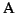for S.

Scoring Pairwise Sequence Alignments To determine if two sequencesare related and have a common ancestor the sequences are usually aligned, and the problem is to find the alignment that maximizes the probability that the two sequences are related: To actually calculate these probabilities, one applies a Markovian model for sequence evolution [25,3]. This begins with an alignment of the two sequences, e.g.

VNRLQQNIVSL____________EVDHKVANYKP
VNRLQQSIVSLRDAFNDGTKLLEELDHRVLNYKP

The gaps arise from insertions (or their counterpart deletions) during divergent evolution. The alignment is normally done by a dynamic programming (DP) algorithm using Dayhoff matrices [12,32,28,1], which finds the alignment that maximizes the probability that the two sequences evolved from an ancestral sequence as opposed to being random sequences. An affine gap cost is used according to the formula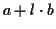, where a is a fixed gap cost, l is the length of the gap and b is the incremental cost [1,4]. More precisely, we are comparing two possibilities
a)
that the two sequences arose independently of each other (implying that the alignment is entirely arbitrary, with amino acid i in one protein being aligned to amino acid j in the other is occurring no more frequently than expected by chance, which is equal to the product of the individual frequencies with which amino acids i and j occur in the database)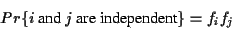(1)

b)
that the two sequences have evolved from some common ancestral sequence after t units of evolution where t is measured in PAM units .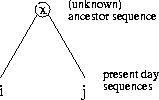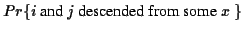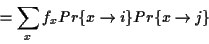(2)

Definition 0.5   The score of an optimal pairwise alignment OPA(s1, s2) of two sequences s1, s2 is the score of an alignment with the maximum score where a probabilistic scoring method [6,10] is used. We refer to a pairwise alignment of two sequences s1, s2 with.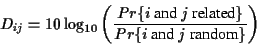(3)

The entries of the Dayhoff matrix are the logarithm of the quotient of these two probabilities. Note that scores represent the probabilities that the two sequences have a common ancestor. The larger the score is the more likely it is that the two sequences are homologous and therefore have a common ancestor. Related work There are two main methods for finding an optimal MSA:
• global methods which construct an alignment throughout the length of the entire sequence (see below), and
• local methods which only attempt to identify an ordered series of motifs (homologous regions) while ignoring regions between motifs [17,18].
We are concerned with global methods and we briefly describe the different strategies used along with some of their drawbacks. HeuristicsNext: Progressive Alignment:
Chantal Korostensky
1999-07-14